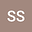Conservation laws and exact solutions for the generalized Ostrovsky equation using symmetry analysis
•## Abstract

In this work we consider a generalized Ostrovsky equation depending on two arbitrary functions and we make an in-depth study of this equation. We obtain the Lie symmetries which are admitted by this equation and some exact solutions as a periodic or solitary waves, obtained through ordinary and partial differential equations. Also, by means of the concept of multiplier, we obtain a wide range of conservation laws which preserve properties of the generalized Ostrovsky equation.
10 Jul 2021Submitted to Mathematical Methods in the Applied Sciences
11 Jul 2021Submission Checks Completed
11 Jul 2021Assigned to Editor
20 Jul 2021Reviewer(s) Assigned
01 Dec 2021Review(s) Completed, Editorial Evaluation Pending
02 Dec 2021Editorial Decision: Revise Major